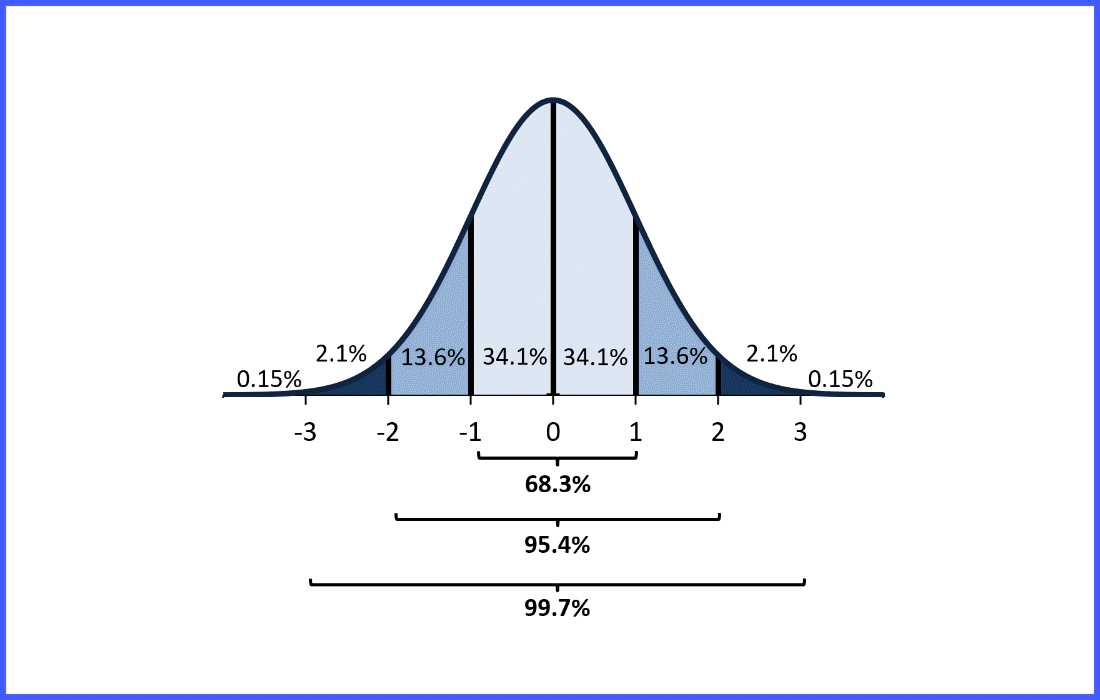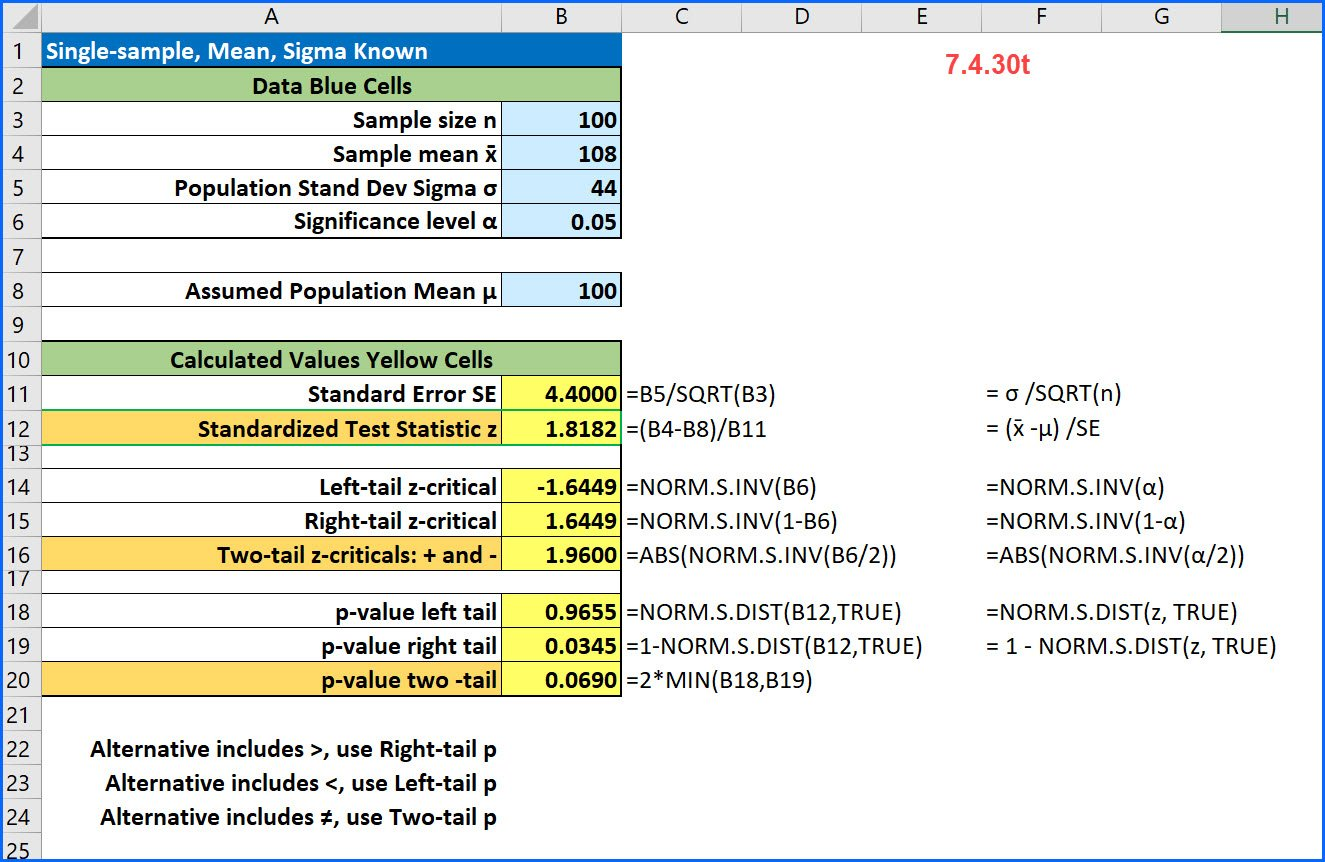# BUS 503

Posts related to my Excelsior BUS 503 Quantitative Methods course.## Empirical Rule and z-score Probability

Empirical Rule The Empirical Rule applies to a normal, bell-shaped curve than is symmetrical about the mean. It states that within one standard deviation of the mean (both left-side and right-side) there is about 68% of the data; within two standard deviations of the mean (both left-side and right-side) there is about 95% of the data; …## Single-sample z-test for the mean [7.4.30t]

Here is a common problem from intro stats: [7.4.30t] A random sample of 100 observations from a population with a standard deviation of 44 yielded a sample mean of 108. Test the null hypothesis that μ = 100 against the alternative that μ > 100 at an alpha of 0.05. Here because the alternative contains …